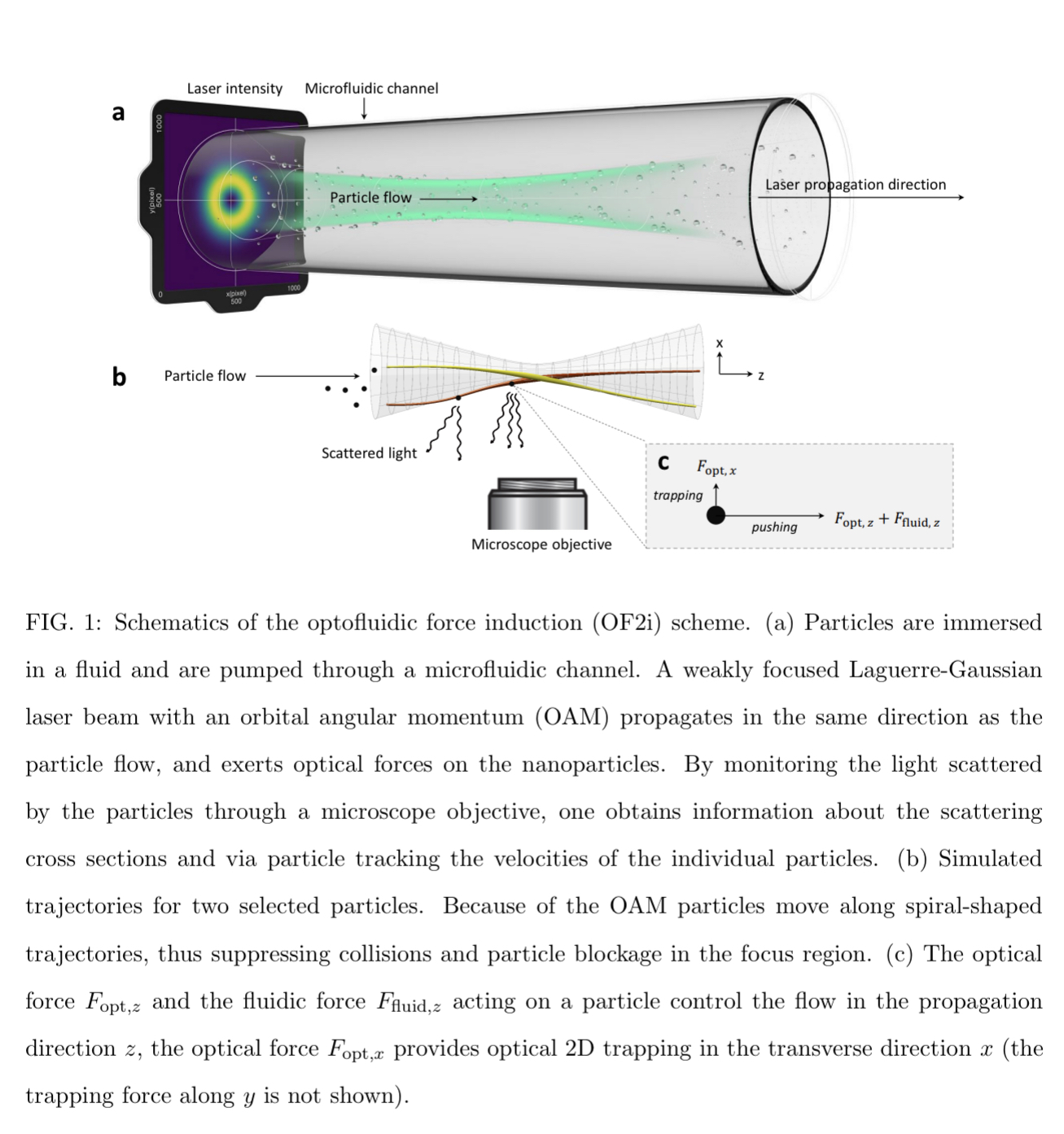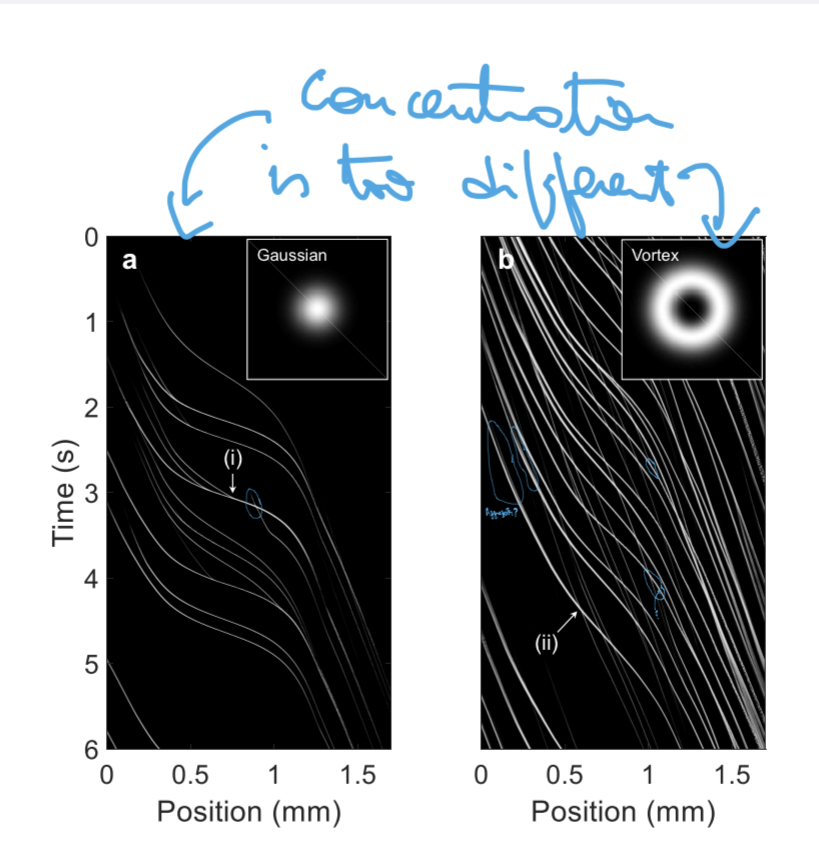# 202304271736

First published:

Last Edited:

Number of edits:The OF2i principle (commercialized by Brave Analytics ) is based on optically trapping particles as they move through a channel. Using a gaussian beam would be possible, but it will also induce particle to bounce into each other in the center of the beam. That is why the authors opted for using a vortex-beam, generated with a phase-mask. Since the beam has a non-zero angular momentum, particles are "trapped" in one direction and forced to rotate as they move along the channel.

If the optical force compensates the force exerted by the fluid, the method is called optical chromatography .

In this paper, the forces are applied in the same direction than the flow, which means particles are accelerated as they travel through the channel. This allows to study around 4000 particles per minute. In order to trap the particles, they use a 532nm laser at 2W . The channel has a diameter of 1mm. The microscope uses a 10X objective, which yields $1.18\mu \textrm{m/px}$ .

To extract information about the particles, the authors use the following equation:

$$v(r) = v_{\textrm{fluid}} + \frac{F_\textrm{opt}(r)}{6\pi\eta R }$$

And they use a model to calculate $F_\textrm{opt}(r)$ that should have a limited number of parameters (but this model is superficially explained, and it is based on Mie-theory). It is important to point out that the refractive index of the particles is one of the inputs .The results look like the image above. The speed can be calculated by the derivative of the trajectory in the kymographs and then the minimum (or maximum) velocity can be calculated and feed to the equation above.

What I don't understand is how are they dealing with particles out of focus . Especially on the right image, where particles should be following a spiral trajectory. It is clear there are bright particles and dim particles overlapping and not collisioning (like point ii) but I have marked some cases of doublets, or particles that seem to aggregate 1 .

Moreover, the authors claim they can study 4000 particles/minute, but the images above show around 10 particles entering the FOV in the 6-second time span, which means around 100 particles/minute. If the concentration would increase 40-fold, I wonder how will they cope with the amount of crossings and overlaps.

1. According to the authors, the particles deviate only slightly from the center of the capillary, and therefore they are never out of focus (and they are always exposed to the maximum velocity profile of the flow inside the channel.)

These are the other notes that link to this one.